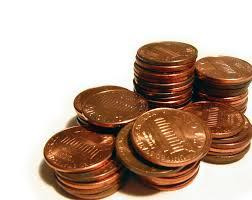# Cents

Julka has 3 cents more than Hugo. Together they have 27 cents. How many cents has Julka and how many Hugo?

j =  15
h =  12

### Step-by-step explanation:

j=3+h
j+h=27

h-j = -3
h+j = 27

h = 12
j = 15

Our linear equations calculator calculates it.Did you find an error or inaccuracy? Feel free to write us. Thank you!Tips to related online calculators
Do you have a system of equations and looking for calculator system of linear equations?